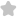3. A man is slipping on a frictionl...
Question# 3. A man is slipping on a frictionless inclined plane & a bag falls down from the same height. Then the speed of both is related as (1) V. >Vm (2) V, IIT/JEE Physics SolutionShare1014.0 (1 ratings)velocity vector of bag ( =g t bar{j} ) velocity vector of man ( =g sin theta t ) ( v_{m}=g sin theta t ) ( sqrt{b}=g t ) ( sin theta quad ) varies from (1,1) max possible ( v ) ane ( =1 ) only if ( theta=90 ) but inclined ( ] ) ( theta<90 ) ( left.therefore quad V_{text {bag }}rightrangle sqrt{operatorname{mon}} )1014.0 (1 ratings) Rate SolutionShareQuick and Stepwise Solutions Just click and SendOVER 20 LAKH QUESTIONS ANSWERED Download App for Free